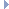# OpenGraph DrawingFramework

v. 2022.02 (Dogwood)

ogdf::SimDrawCreator Class Reference

Creates variety of possible SimDraw creations. More...

#include <ogdf/simultaneous/SimDrawCreator.h>Inheritance diagram for ogdf::SimDrawCreator:

## Public Member Functions

SimDrawCreator (SimDraw &SD)
constructor More...

void clearESG ()
clears edgeSubGraphs value More...

void createRandom (int numberOfNodes, int numberOfEdges, int numberOfBasicGraphs)
randomly creates a simdraw instance More...

void randomESG (int graphNumber)
randomly chose edgeSubGraphs value for graphNumber graphs More...

void randomESG2 (int doubleESGProbability=50)
randomly chose edgeSubGraphs value for two graphs More...

void randomESG3 (int doubleESGProbability=50, int tripleESGProbability=25)
randomly chose edgeSubGraphs value for three graphs More...

uint32_t & SubGraphBits (edge e)
returns SubGraphBits from edge e More...

uint32_t & SubGraphBits (edge e) const
returns SubGraphBits from edge e More...Public Member Functions inherited from ogdf::SimDrawManipulatorModule
SimDrawManipulatorModule ()
default constructor More...

SimDrawManipulatorModule (SimDraw &SD)
constructor More...

const SimDrawconstSimDraw () const
returns base instance More...

void init (SimDraw &SD)
initializing base instance More...Protected Attributes inherited from ogdf::SimDrawManipulatorModule
Graphm_G
pointer to current graph More...

GraphAttributesm_GA
pointer to current graphattributes More...

SimDrawm_SD
pointer to current simdraw instance More...

## Detailed Description

Creates variety of possible SimDraw creations.

This class is used for creating simdraw instances. Possible features include reading a graph, randomly modifying or clearing the edgeSubgraph value and changing the subGraphBits.

Definition at line 45 of file SimDrawCreator.h.

## ◆ SimDrawCreator()

 ogdf::SimDrawCreator::SimDrawCreator ( SimDraw & SD )
inlineexplicit

constructor

Definition at line 50 of file SimDrawCreator.h.

## ◆ clearESG()

 void ogdf::SimDrawCreator::clearESG ( )

clears edgeSubGraphs value

This method clears all SubGraph values from m_G. After this function all edges belong to no basic graph. CAUTION: All edges need to be reset their edgeSubGraphs value for maintaining consistency.

## ◆ createRandom()

 void ogdf::SimDrawCreator::createRandom ( int numberOfNodes, int numberOfEdges, int numberOfBasicGraphs )

randomly creates a simdraw instance

This method creates a random graph with numberOfNodes nodes, numberOfEdges edges. It is transfered into a simdraw instance with numberOfBasicGraphs basic graphs.

randomSimpleGraph from graph_generators.h is used to create a random graph. Furthermore randomESG is used on this graph to generate numberOfBasicGraphs basic graphs.

## ◆ randomESG()

 void ogdf::SimDrawCreator::randomESG ( int graphNumber )

randomly chose edgeSubGraphs value for graphNumber graphs

Assigns random edgeSubGraphs values to all edges to create a SimDraw instance consisting of graphNumber basic graphs. Each edge has an equal chance for each SubGraphBit - value.

## ◆ randomESG2()

 void ogdf::SimDrawCreator::randomESG2 ( int doubleESGProbability = 50 )

randomly chose edgeSubGraphs value for two graphs

Assigns random edgeSubGraphs values to all edges to create a SimDraw instance consisting of two basic graphs. Each edge in m_G has a chance of doubleESGProbability (in Percent) to belong to two SubGraphs. Otherwise it has equal chance to belong to either basic graph.

## ◆ randomESG3()

 void ogdf::SimDrawCreator::randomESG3 ( int doubleESGProbability = 50, int tripleESGProbability = 25 )

randomly chose edgeSubGraphs value for three graphs

Assigns random edgeSubGraphs values to all edges to create a SimDraw instance consisting of three basic graphs. Each edge in m_G has a chance of doubleESGProbabilit (in Percent) to belong to two basic graphs and a chance of tripleESGProbability (in Percent) to belong to three basic graphs.

 void ogdf::SimDrawCreator::readGraph ( const Graph & G )
inline

Definition at line 59 of file SimDrawCreator.h.

## ◆ SubGraphBits() [1/2]

 uint32_t& ogdf::SimDrawCreator::SubGraphBits ( edge e )
inline

returns SubGraphBits from edge e

Definition at line 53 of file SimDrawCreator.h.

## ◆ SubGraphBits() [2/2]

 uint32_t& ogdf::SimDrawCreator::SubGraphBits ( edge e ) const
inline

returns SubGraphBits from edge e

Definition at line 56 of file SimDrawCreator.h.

The documentation for this class was generated from the following file: